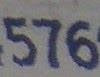## Wednesday, March 2, 2011

### 576

576 = 26 x 32.

576 has a unique representation as a sum of three squares: 576 = 82 + 162 + 162.

576 is the number of 4 x 4 Latin squares.

576 is the smallest square such that it remains a square when its leading digit is increased by 1: 676 = 262.

576 = (2 + 3 + 5 + 7 + 11)2 - (22 + 32 + 52 + 72 + 112).New Balance produces a 576 line of fitness walking shoes.